﻿ InnovateFPGA | Americas | EM031 - Real-time video frames classification based on MobileNet convolutional neural net
Annual: 2019

📁Machine Learning
👤Alexander Kustov
(IPPM RAS)
📅Oct 06, 2019👀 5331   💬 2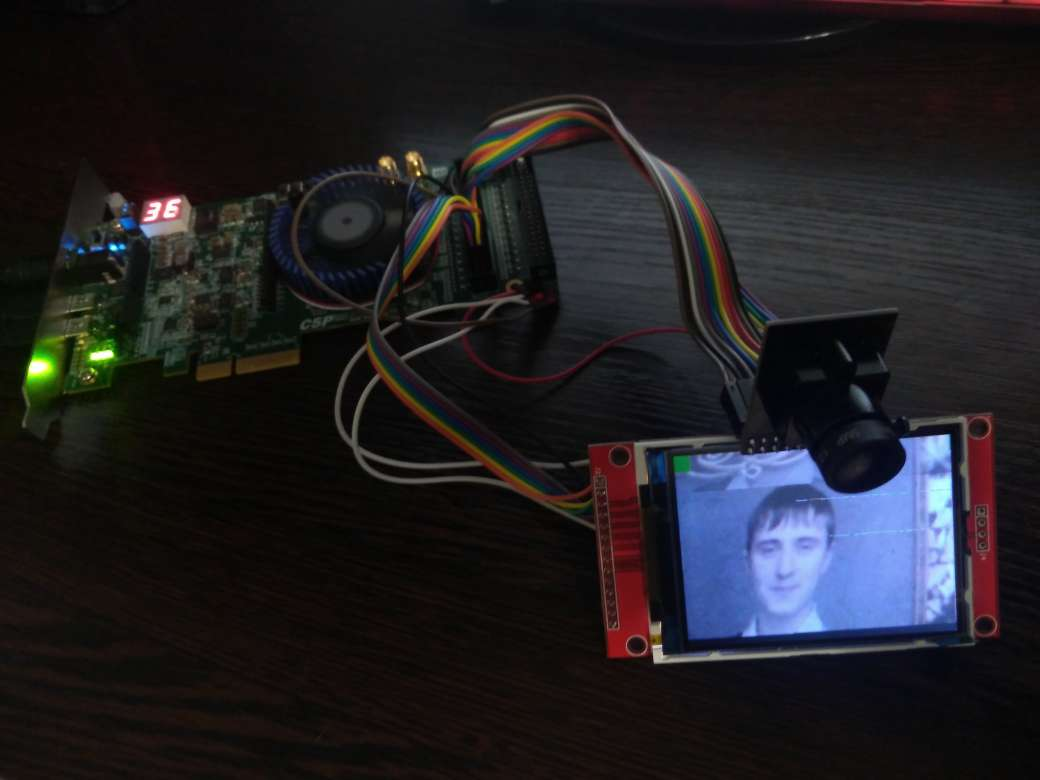EM031 » Real-time video frames classification based on MobileNet convolutional neural net

### Description

Neural networks have become a popular means of solving problems that are difficult to access for ordinary algorithms. Neural networks are used in robotics, medicine, Internet, business, geological exploration, and other fields. The present work is dedicated to the use of complex neural networks in FPGA for solving the complex task of processing a video stream and detecting objects of various kinds in the frame. As an example, it is planned to use a neural network based on MobileNet_v1. The prototype will recognize the presence of people in the frame. It is also planned to add several alternative models with high detection accuracy (recognition of cars and animals in the frame).

### Project Proposal

#### 1. High-level Project Description

The proposed project works on the basis of the OpenVINO debug board, and camera and screen that are connected to it. This device can recognize presence of a certain type of objects in a camera frame. Qualification takes place in real time, that is, each and every frame is processed. As to neural networks, we have chosen MobileNet (v1) to solve this task, because it has simple structure, high performance and sufficient qualification accuracy.

### Usage:

For project realization, we have created semiautomatic flow, which includes such steps as preparing images for neural network training, actual training process, model transformation and scaling, floating-to-fixed point conversion with automatic choice of dimensions of weights and feature maps. And, finally, we have created code to generate Verilog description of neural network that can be flashed into FPGA. We have uploaded several ready project files to be flashed for such typical tasks as detecting people, cars or animals in the frame. Peripheral connection scheme is also provided. That is, to obtain a workable device, one only needs to connect the necessary components according to the specified scheme and flash a ready project.

### Users:

The product will be of interest to consumers who need a standalone device with the ability to recognize some type of objects (e.g. people, cars, animals) in real time mode independently of any PC or server (in places where there is no access to powerful servers). Note that the device has low cost, very high speed and low power consumption. In addition, students and gadgeteers can also take interest in this project. The proposed flow is universal enough; it contains all design steps from preparing image dataset for training to creating Verilog code of the resulting device. This code can serve as base for creating similar units with some changes, for example, detecting different types of objects generated with another dataset, or using another FPGA, or another camera, etc. The chosen network is comparatively easy to implemented, that is, the hardware description is easy to understand.

#### 2. Block Diagram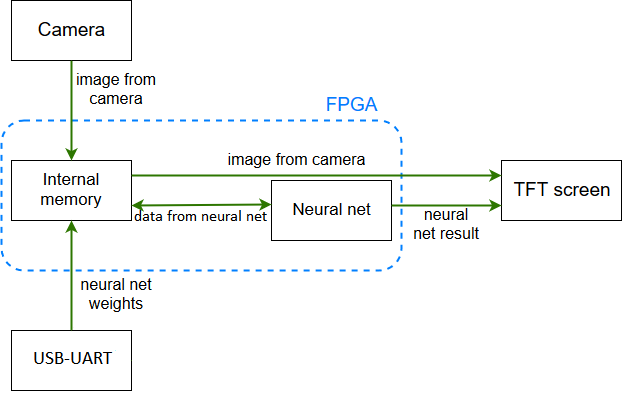#### 3. Intel FPGA Virtues in Your Project

1. The use of FPGA (due to the large computing power) allows implementing a device that can process each frame of video stream in real time.

2. FPGA has low power consumption and high processing speed which makes it possible to realize high-grade neural network of high accuracy that can work in a portable device from a battery without additional power sources.

3. Large amount of internal memory makes it easy to store and update the weights that are used to calculate neural networks in FPGA.

4. Using FPGA makes it possible to directly connect external peripherals such as camera and screen.

5. One can rewrite just the weights of the neural network to use a universal device for solving a large number of different tasks.

6. Transition from floating point to fixed point calculations reduces the amount of memory required for storing the weights and also increases device speed.

7. The HPS core ensures convenient connection to WiFi and Ethernet to communicate with external server. This allows sending the results of object detection to a central server, if necessary.

## Characteristics:

1. We have currently implemented a semi-automatic Verilog description generator from high-level code (Keras/Tensorflow). We plan to make it fully automatic.

2. To speed up calculations, we switched to calculations with fixed point and implemented the device at the hardware level (without using NIOS). Our device description is made with Verilog HDL only.

3. The developed device can be easily reprogrammed to detect any other type of objects: it is enough to replace the model weights file at the time of device initialization.

4. Usage of the well-known neural network architecture, as well as the availability of code to train it on an arbitrary data set, allows the device to be used by enthusiasts and easily reprogrammed to fit their tasks. Project is open source and available at GitHub:
https://github.com/ZFTurbo/MobileNet-in-FPGA

Video demonstration of the prototype :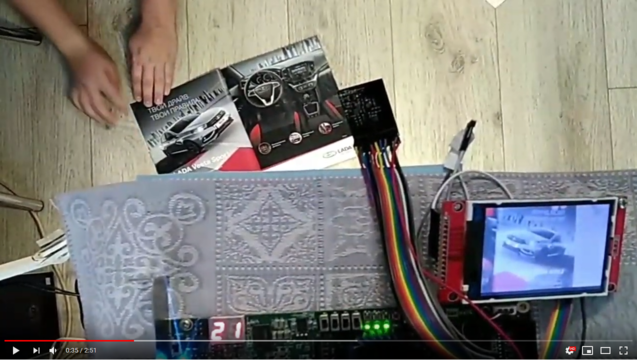## MobileNet scheme

The scheme is implemented for the MobileNet version with input of 128x128 pixels and alpha = 0.25 (see figure below). In this scheme, there are no BatchNormalization blocks, since they are combined with Conv2D layers due to the operation of BatchNorm fusion. The last Fully-Connected layer contains two neurons: one is responsible for the presence of an object in the frame, the other – for its absence.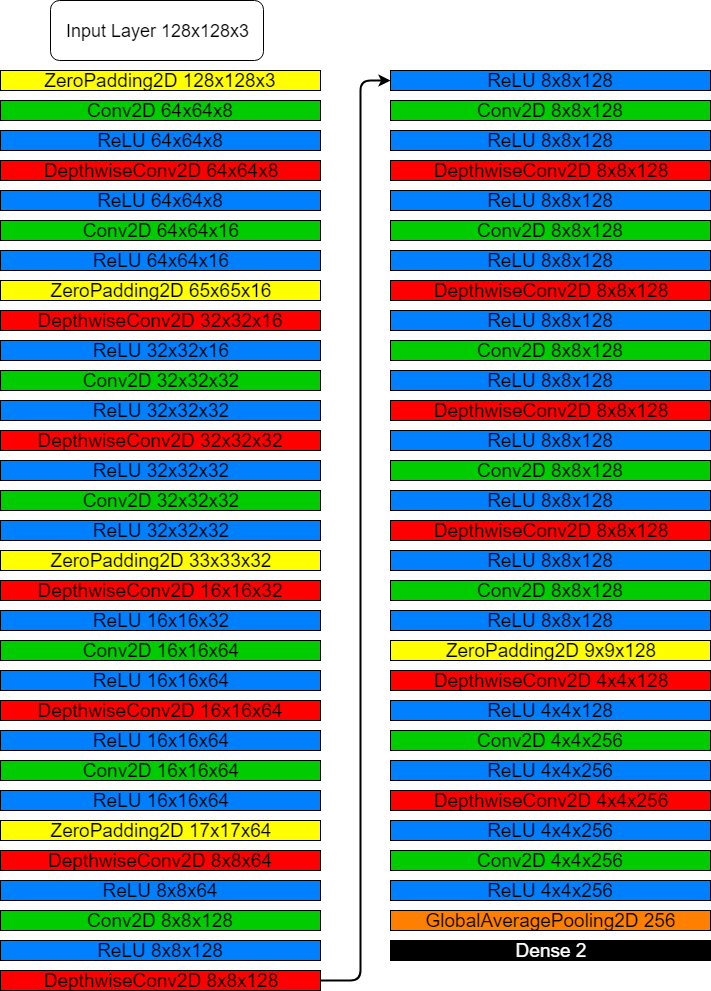## BatchNorm Fusion

If the Batch Normalization layer is placed right after the convolutional block (this is how it is located in most modern neural networks), then it can be removed by recalculating the weights of the convolutional layer using the formulas below: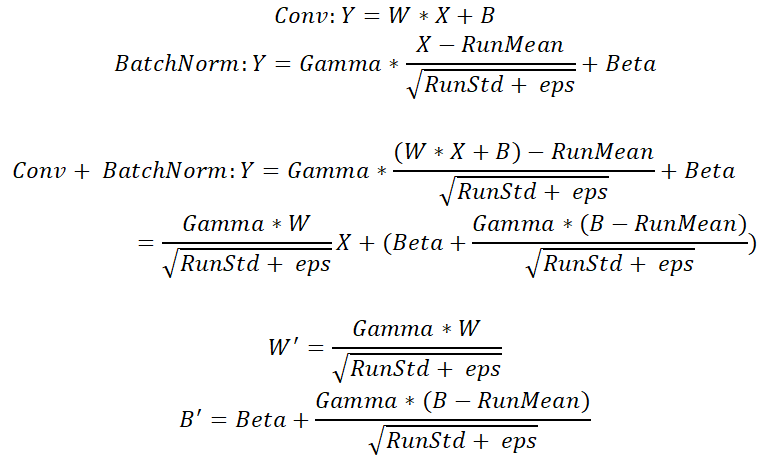## Transition to fixed-point calculations

Consider the first layer of a neural network of Convolution type – convolution block, which is the basic one in most convolutional neural networks.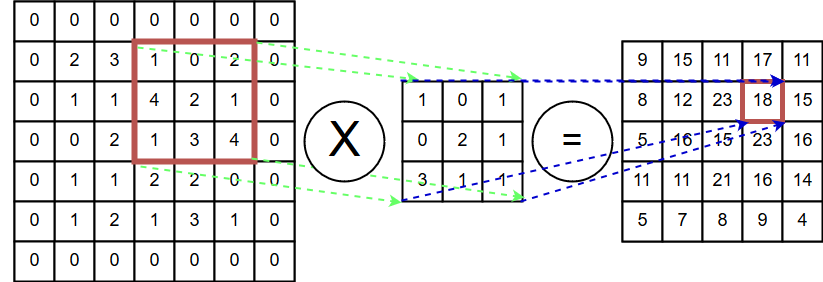At the input of the layer, there is a two-dimensional matrix (an original image), the values of which are on the interval [0; 1].

It is also known that if a∈[-1;1] and b ∈[-1;1], then a*b ∈ [-1;1].

Since the weights and biases are known, it is possible to calculate the potential minimum and maximum in the second layer. If we divide the weights and biases by the maximum value, then we can guarantee that for any configuration of the input data the value on the second layer will not exceed 1. We call this value the reduction coefficient of the layer. The situation at the second layer is almost the same, namely: at the input of the layer, the value is from the interval [-1; 1]; so the reasoning can be repeated.

It can be easily shown that for a neural network, at the last layer (after all weight reductions), the position of maximum on the last neuron does not change, that is, the neural network works equivalently to the neural network without reduction from the point of view of floating-point calculations.

After performing reduction on each layer, we can move from floating-point to fixed-point calculations, since we know the exact range of values at each stage of the calculations.

When we use fixed-point calculations with limited bit width of weights and intermediate results, rounding errors are inevitable. Such errors tend to accumulate from layer to layer, which can lead to incorrect operation of neural network. Note that we consider the operation incorrect if the qualification result does not match mathematical model rather than the real answer. To check correctness, we have to run both floating-point mathematical model and fixed-point mathematical model (or Verilog testbench) for all test images and to compare the network output. Ratio of non-matching answers to the number of tests is the measure of failures for the given dimension of weights and biases. When choosing bit width, we can focus on dimension values, for which failure rate equals zero.

To go to calculations with fixed point, it is necessary to determine how many bits are enough to keep calculation accuracy, that is, the classifier should produce the same results in floating-point and fixed-point calculations (see the figure).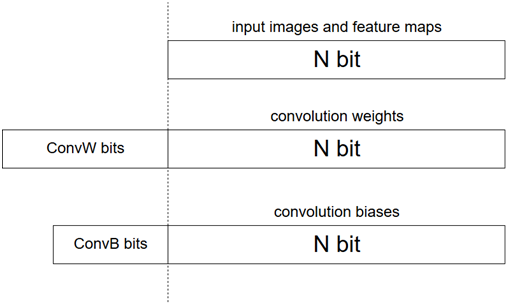We can slightly complicate the task and find the optimal dimensions separately for feature maps, weights, and biases (see the figure).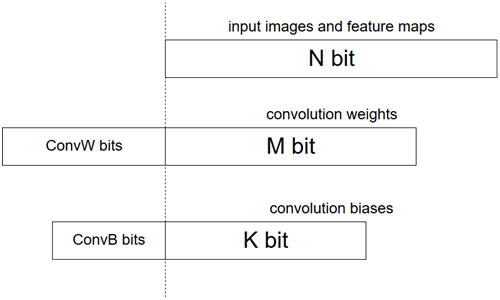We can use the following algorithm to choose bit width. Set the variable N to some large value on which the network will certainly work accurately. Then pass all the validation images through the network and compare the classification results with a floating point mathematical model. If the accuracy is higher than the required one, reduce N. Then try to separately reduce the dimensions of weights M and biases K until the optimum is found.

MobileNet (v1) uses activation function RELU(6) with value 6, which is not appropriate, we can change it to value 1, then the values of feature maps should be normalized after each activation at interval [0; 1] exactly as for the input image. It is easy to do: we just need to divide all first layer values by 6, then divide shifts at each layer by 6 and replace each instance of RELU(6) to RELU(1). Position of maximum at the last layer will not change, that is, qualification will be the same as for the initial model.

At this stage, we normalized the whole input image and all biases to interval [0; 1]. However, weights and biases can exceed 1. Thus, we have to find maximum and minimum for these values to define how many additional bits should be stored. The following formulas can be used: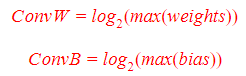If the network is trained at big data set and has good qualification accuracy, ConvW and ConvB will not be too big. Actually, we got values ConvW = 7 and ConvB = 3 for all our test networks.

Image resizing

Image obtained from camera has resolution of 320х240. But neural net uses images of size 128x128 and 3 channels. Image is resized to 128x128 pixels using bilinear interpolation. We use 4 neighboring pixels and find new one. Since size of input image and output images are known, coefficients are fixed and conversion becomes very simple.

Processing image with Neural Net

MobileNet_v1 neural net uses 3 different types of convolutional layers: Convolution2D (3x3), DepthwiseConv2D and Convolution2D (1x1). Each layer has its own shape of input feature maps and set of weights. Typical shape of input data of each layer is WxHxC – where W is width, H – height and C – number of feature maps. Layers that are far from input have smaller W and H but larger C value. Weights have shapes like IxOxLxM, where I is the number of feature maps on layer inputs, O – number of feature maps on output of layer, L and M – kernel size. L = M = 3 for Convolution2D (3x3) and DepthwiseConv2D, and L = M = 1 for Convolution2D (1x1). All these parameters for each layer are stored in main Verilog module with neural net implementation. Also module contains information about the address where layer input data is stored, address where we need to store layer output, address of weights, layer type and some other data.

Let us describe all types of layers.

1) Convolution2D (3x3) layer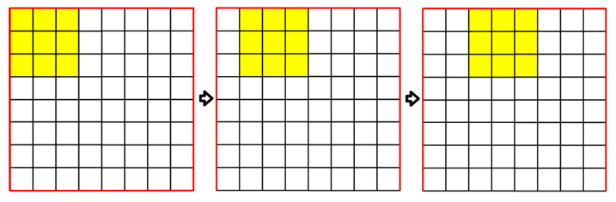3x3 kernel is moving along feature map calculating new pixel in output feature map. Kernel weights change when we switch to another input feature map. Formula to calculate output pixels is as follows:

Pnew=(p1,1w1,1+p2,1w2,1+p3,1w3,1+p4,1w4,1+p5,1w5,1+p6,1w6,1+p7,1w7,1+p8,1w8,1+p9,1w9,1)+…+(p1,nw1,n+p2,nw2,n+p3,nw3,n+p4,nw4,n+p5,nw5,n+p6,nw6,n+p7,nw7,n+p8,nw8,n+p9,nw9,n)+bias,

where p is pixel value, w – weight, n – number of input feature maps, bias – bias value.

2) Depthwise2D (3x3) layer

This layer is a simplified version of standard Convolution2D block, where number of input feature maps is the same as number of output feature maps and for each input feature map only one set of weights is used. So formula becomes simpler:

Pnew=(p1w1+p2w2+p3w3+p4w4+p5w5+p6w6+p7w7+p8w8+p9w9)+bias

3) Convolution2D (1x1) layer

In this type of layer we use only one pixel instead of nine in 3x3 version.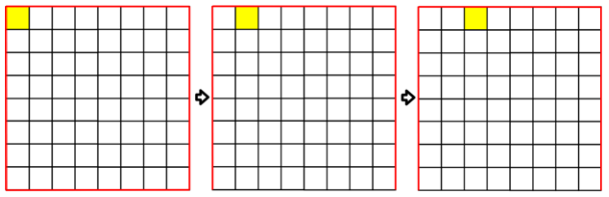Formula has the following form:

Pnew=(p1w1+p2w2+...+pnwn)+bias

where n equals to number of input feature maps.

4) Dense (Fully-Connected) layer

This layer is used for the final classification result. It outputs probabilities of the events: the initial image contains “human” object or not. Its connection scheme is as follows: all inputs of the layer are connected to all outputs of the layer.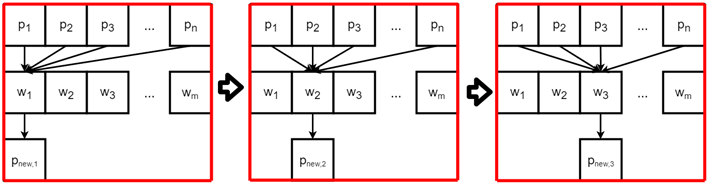Formula for the layer is as follows:

Pnew=(p1w+p2w+...+pnw)+bias,

where n is the number of feature maps.

For Verilog implementation, some layers are excluded being replaced by layer functions that are also listed in the main network module as parameters for each layer. So, ZeroPadding2D, which adds zeroes around every input image (see Fig.), is replaced by adding a function to the next layer; this function detects whether the current pixel is at the image border; if yes, to which exactly border or borders it belongs. These options are used for calculating new pixel of the current layer.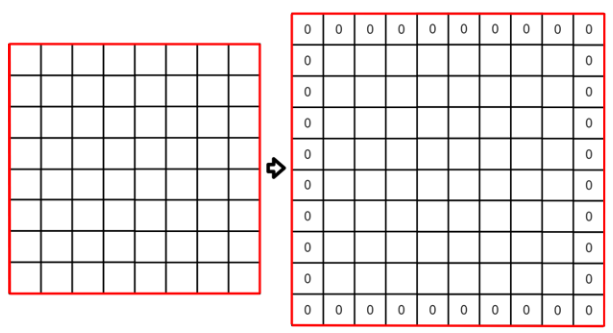ReLU(0, 1) is the activation function, whose graph is shown in the following figure.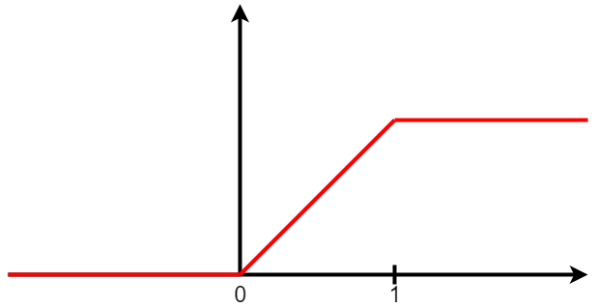Fig. ReLU.

In hardware implementation, the function is implemented as the function of the previous convolutional layer, which compares the output value with the minimum and maximum values, i.e. with 0 and 1. If this value is less than zero, then the resulting value is replaced by zero, and if it exceeds one, then output is one.

GlobalAveragePooling2D is the layer that calculates average value for each input picture. In hardware implementation, this layer is replaced by the function of the previous layer, which finds average value for each output picture immediately during the layer execution.

In addition to the listed layer functions, in the hardware implementation, there is stride function that determines how many pixels at a time are shifted in convolutional layers. This function is shown in Fig. for different stride values.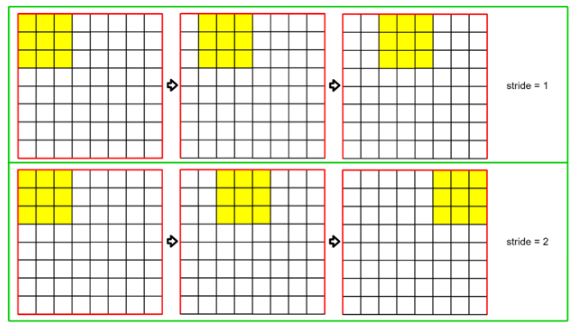## Convolution modules

Convolution module is a hardware module that performs multiplication and addition of input values of image pixels and weight coefficients. For the presented hardware implementation, we use convolutional modules that work according to the formula:

Pnew=p1w1+p2w2+p3w3

There is a good reason to choose this type of convolutional module for hardware implementation. The fact is that the more mathematical operations, multiplication especially, are performed in one cycle, the greater is the delay, which affects FPS. Therefore, the minimal number of 3 multiplications is chosen. Also, this decision helps saving FPGA resources.

To speed up calculations, several parallel convolutional modules are used. In this case, performance increases in proportion to the number of convolutional blocks.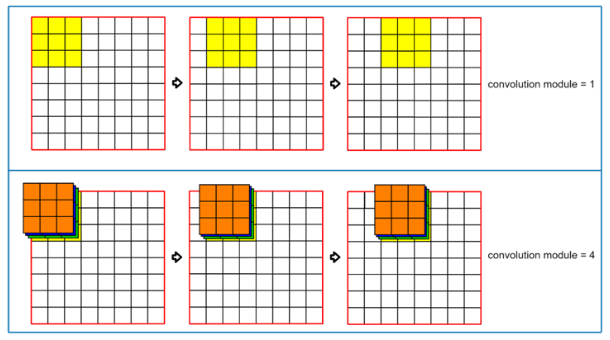For comparison, several hardware implementations with different number of convolutional modules and multiplications were created: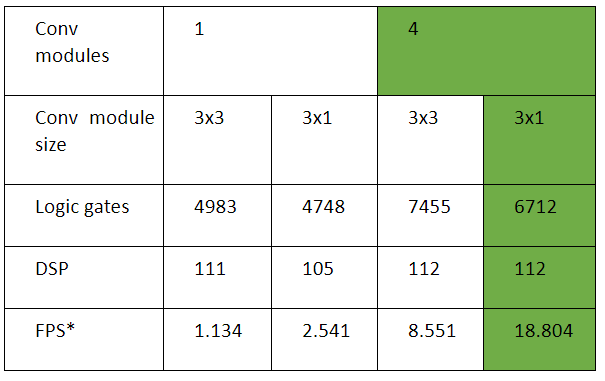*- provided that the network clock domain and internal memory clock domain work at the same frequency. For each implementation, calculations were performed at the maximum possible frequency.

In this work we have presented implementation highlighted in green, since it has the best characteristics.

Shifting of neural network results

Each layer of the neural network receives input data of a certain size. Data resulting from operations performed on the previous layer require more bits. Thus, the bit width should be reduced to acceptable. We do reduction by shifting data as shown in the example for prime numbers (see Fig. below).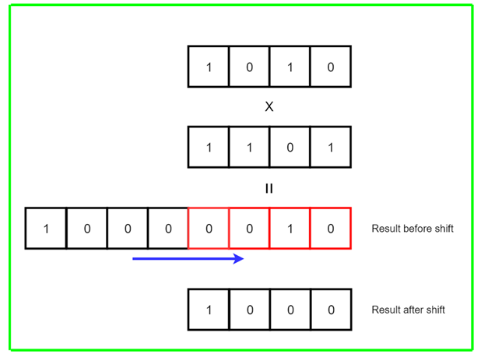To get more accurate result, shifting is carried out after the entire layer is completed.

## Hardware implementation of RAM memory

We have to store input and output images, weights and biases. For this purpose, a module was implemented that writes data to FPGA internal memory and reads it when needed.

A 320x240 picture is stored in memory as it comes from the camera for output on the TFT screen. Since a neural network requires a picture of size 128x128, it is obtained from 320x240 using bipolar interpolation, and also independently stored in memory. This memory area is necessary for the neural network to take a picture at its frequency, without reference to the screen. All the weights necessary for the operation of the neural network also stored in the internal memory, from where they will gradually be called up for certain layers. Four separate memory areas are allocated - for intermediate results before and after the bias application, for weights and for biases. Moreover, the region for intermediate results before the shift is divided in two - for input values ​​of the layer and for the results. After each layer, these two sections of memory change their roles, that is, if initially the first section stores input values, and the second the results, on the next layer, just the opposite, the first sections will store the results, and the second - the input values.

The figure below shows the architecture of internal memory.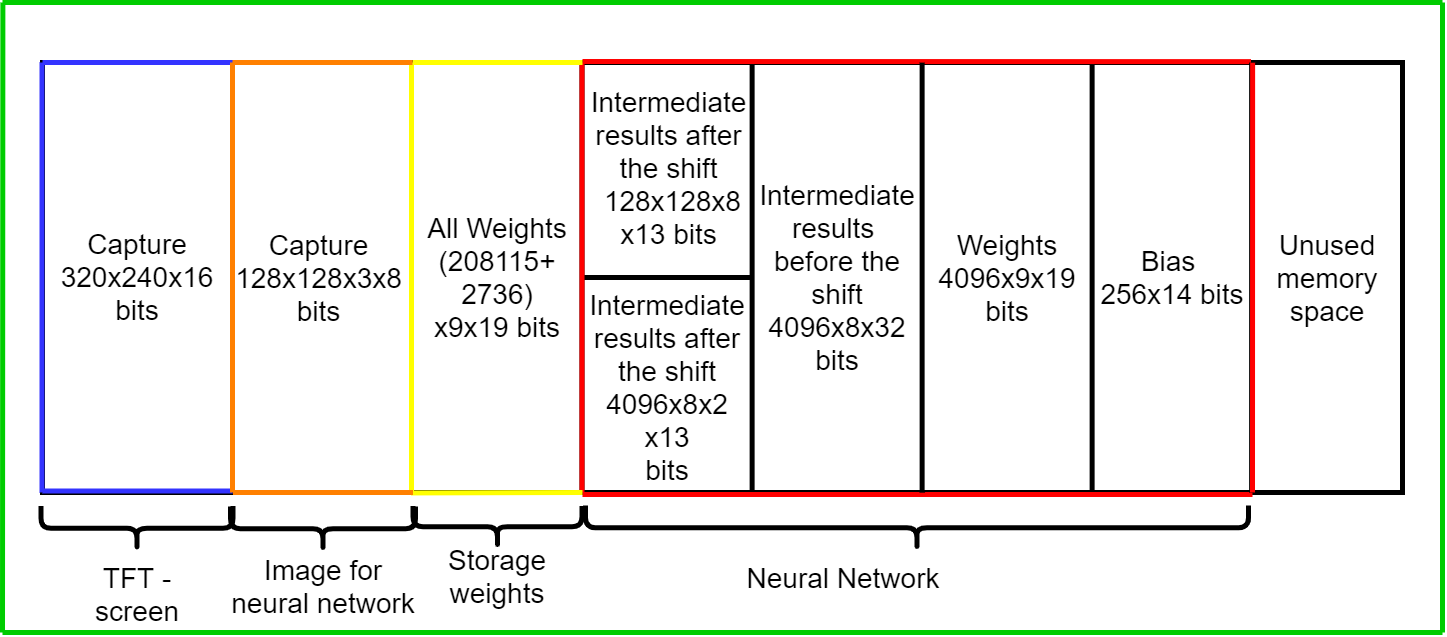For each memory section, we select the minimum number of bits. In this work, there are no more than 256 shifts on the layer, each of which is 17 bits long.

## Variants of device implementation

There are 3 variants of implementing the proposed device in terms of placement in memory:

1. Hardware implementation of the neural network and all intermediate calculations are stored in internal memory, the weights are stored in RAM memory accessible from the Arm kernel. Weights are obtained via HPS bus. We need this variant if there is not enough internal memory to store weights, and there is no memory directly accessible from FPGA. The major disadvantage of this arrangement is slow data exchange between memory and FPGA. A board for this variant: De10-Nano.

2. Hardware implementation of the neural network and all intermediate calculations are stored in internal memory, the weights are stored in RAM memory accessible directly from FPGA. We need this variant if there is not enough internal memory to store weights. The major disadvantage of this arrangement is additional hardware code for memory data exchange and some other extras. A board for this variant: De10-Standard, which has 64 MB RAM memory available from FPGA.

3.  Hardware implementation of the neural network and all intermediate calculations and weights are stored in internal memory. That is, no data exchange with external memory is needed. This realization is the fastest and the easiest to implement. The major disadvantage of this arrangement is that the more internal memory we have, the more is the cost of the board, and also there are heavy restrictions on the size of the neural network. A board for this variant: OpenVINO, which has 13,917 Kbits embedded memory.

## Model preparation

General scheme of preparing neural network model is shown below. At the input of the algorithm, we feed a set of images and their labels (in our case, we support two classes: presence and absence of the object in the frame). As the result of preparation, we immediately get Verilog code to be flashed into FPGA. The algorithm is implemented in Python with Tensorflow and Keras modules and is available at GitHub.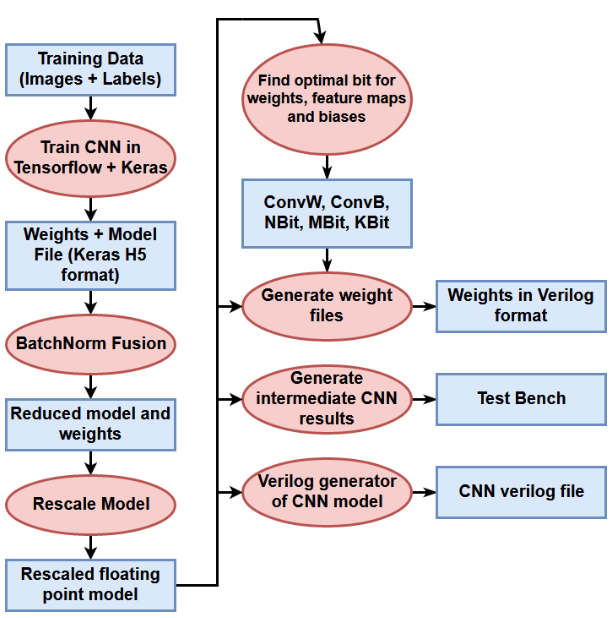For training, we used Open Images Dataset by Google. From the large set of images, we selected images that obviously contain the required class (for people, these are classes: 'Person', 'Man', 'Woman', 'Boy', 'Girl', 'Human body', 'Human eye', 'Skull', 'Human head', 'Human face', 'Human mouth', 'Human ear', 'Human nose', 'Human hair', 'Human hand', 'Human foot', 'Human arm', 'Human leg', 'Human beard'). Such images were labelled as target=1, and images obviously not containing the specified classes were marked as target=0. After reducing size of the initial image, some people become poorly visible. Actually, after decreasing picture to 128 pixels, some images of the required class become less than 5 pixels. For this reason, we mark such images as not containing this class.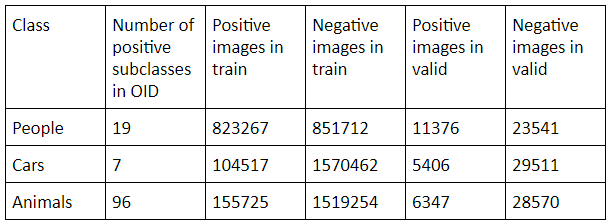Writing weights to memory

The UART port is used to write weights to FPGA memory. The weights are transferred directly from the computer using Python language. To do this, first connect all the necessary wires to OpenVino (see Fig.).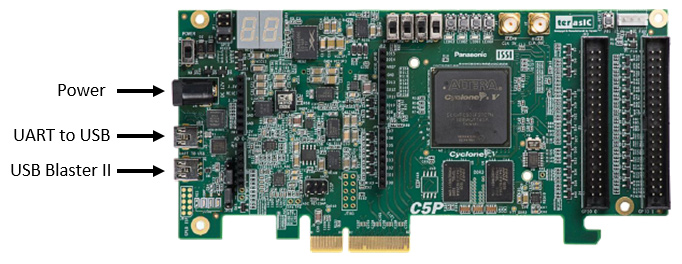Next, turn on the device. Having done this, launch Quartus Prime (we used version 18.0) and flash the entire project using Programmer. Then run the special Python script: utils/data_uart_to_fpga.py. Make sure that your folder with the weight sets is located near the executable file and has correct name. Depending on what set of weights you need – for people, animals or cars – select the appropriate file that should be specified in the code. If everything is done correctly, the job will progress.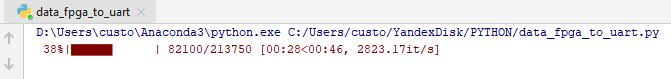Upon completion of loading the weights, an image from the camera will appear on the screen and the neural network will start recognizing it. The result will be displayed in the upper left corner in red (there is an object) or in green (the expected object is absent).

Problems to be solved and Future work

1) Currently, we use too much internal memory because of the specific features of the algorithm for convolutional layers. Thus, we have run out of memory on just 8 convolutional blocks, although the number of occupied LE cells is about 10%. So we can place much more convolutional blocks in FPGA (32 or 64), which will increase performance by times. We plan to do this in the next version of the device.

2) In the current implementation, convolution is performed according to the standard formula, however, there are more efficient implementations of this operation through conversion of convolution by col2im, im2col and DOT-product BLAS operation. Such conversion complicates hardware code of the device greatly, but potentially increases the speed of operation by times.

## Current project compilation results (OpenVino)

LE: 11020/113560 (10%)

Pins: 49/378 (13%)

Memory bits: 9837100/12492800 (79%)

DSP Blocks: 141/342 (41%)

Maximum frequency for neural net (found using Quartus Timing Analysis): 44 MHz

Number of clocks to get the result for single frame (only neural net):  941981

Minimum number of clocks to load image and weights in intermediate memory and calculations (total per single frame):  1208689

Time to process image with neural net (without weights loading time):  941981*(1/(44*106)) = 0.021 sec. Potential number of frames per second 1/0.021 = 47.62.

Number of frames per second overall in current implementation with only 8 convolutional blocks: 41.37 FPS

## Models accuracy

Accuracy of the models after training in Python on validation set from Open Images Dataset is as follows:

MobileNet v1 for classification of people: 84.42%

MobileNet v1 for classification of cars: 96.31%

MobileNet v1 for classification of animals: 89.67%

#### 7. Design Architecture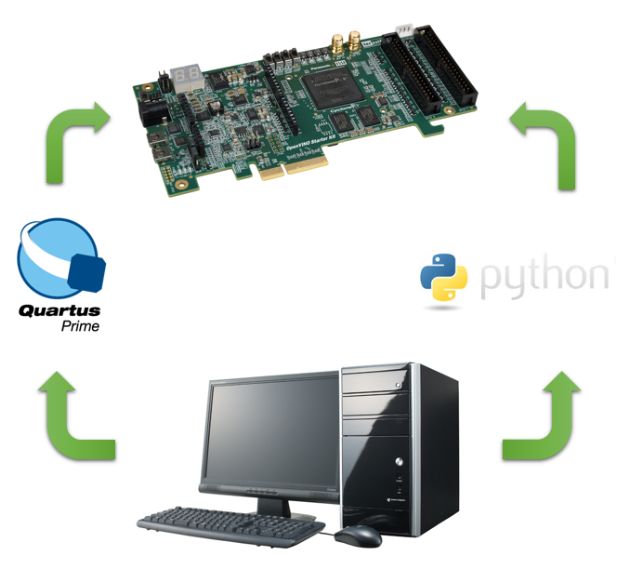Set of peripherals

1) TFT-screen ILI9341 Size: 2.8 ‘’, Resolution: 240x320, Interface: SPI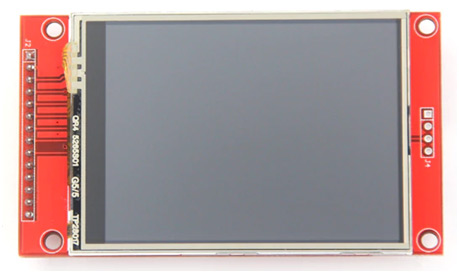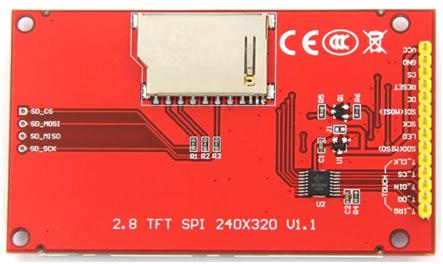2) Camera OV5640. Specification: (5 Mpix) Active array size: 2592 x 1944, output formats: 8-/10-bit RGB RAW output, lens size: 1/4" lens chief ray angle: 24°, input clock frequency: 6~27 MHz, max S/N ratio: 36 dB (maximum) , maximum image transfer rate: QSXGA (2592x1944): 15 fps, 1080p: 30 fps 1280x960: 45 fps, 720p: 60 fps, VGA (640x480): 90 fps, QVGA (320x240): 120 fps.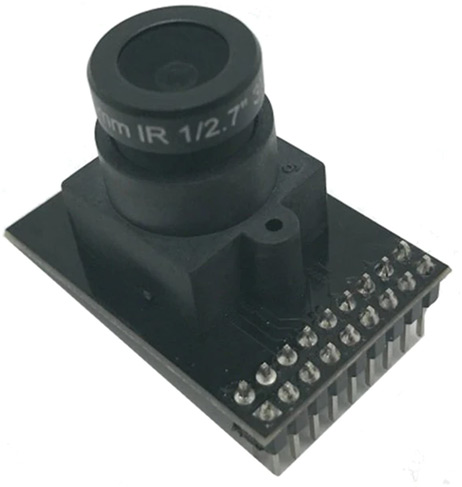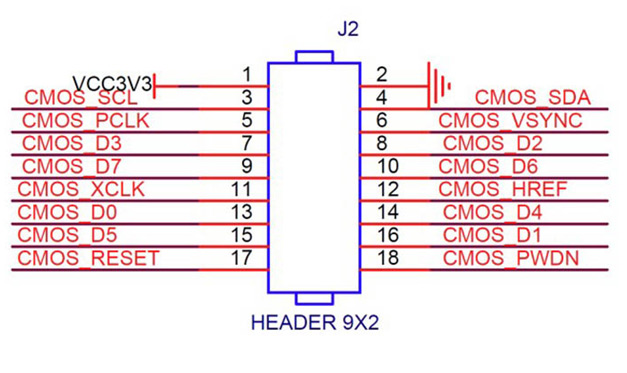3) OpenVINO

13,917 Kbits embedded memory.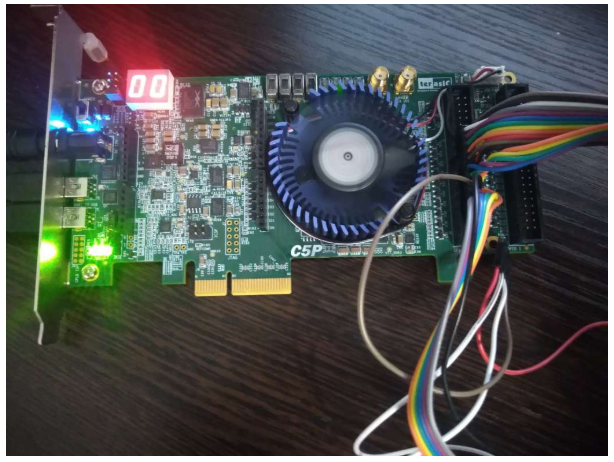4) We also developed a printed board for connecting peripherals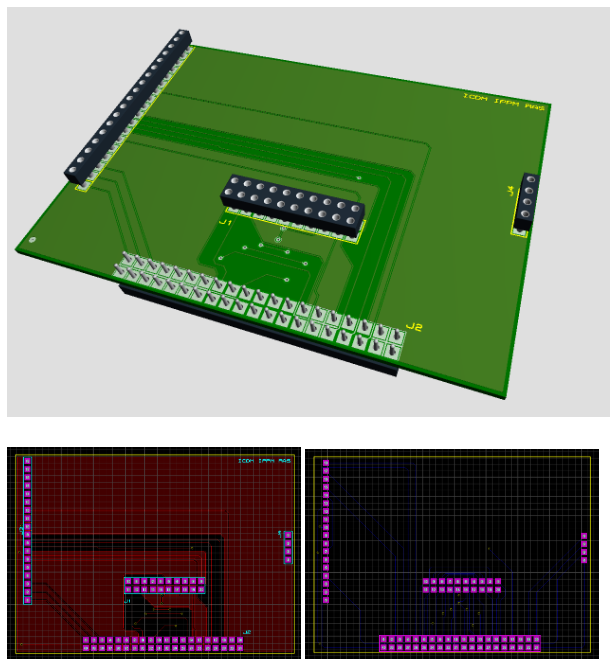Connection of components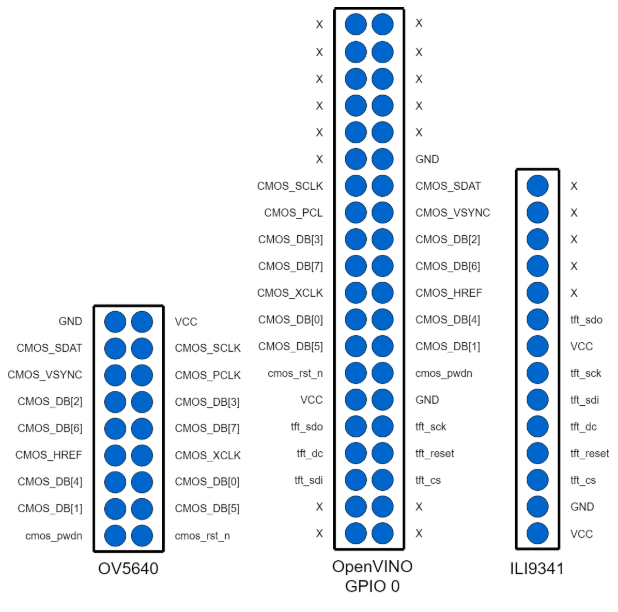## Software implementation of the device

https://github.com/ZFTurbo/MobileNet-in-FPGA

Repository contains:

1. All code needed to train neural net model

2. Code to convert model to fixed point and find optimal bits with minimum loss of detection accuracy

3. Code to convert weights in Verilog format

4. Utils to write weights using UART

5. Code to generate verilog for neural net (using different parameters)

6. Pretrained models (people, cars, animals)

7. Complete Quartus projects for 3 models (people, cars, animals)